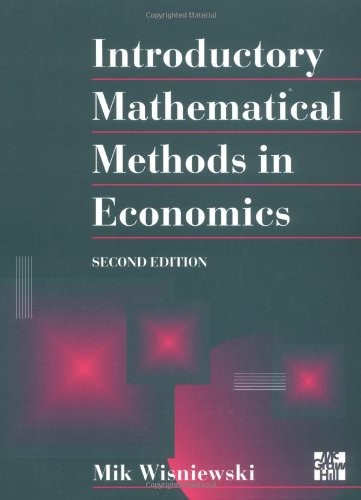# Introductory Mathematical Methods In Economics by Mik Wisniewski

Out of Stock
\$26.49
inc. GST
Introduces undergraduate students studying economics to a useful set of analytical tools and mathematical techniques.
Only 0 left

## Introductory Mathematical Methods In Economics Summary

### Introductory Mathematical Methods In Economics by Mik Wisniewski

Designed specifically for undergraduates taking introductory courses in mathematical economics, this text focuses on the usefulness of mathematical techniques in economics rather than on mathematical theorems and proofs. Allowing students to develop basic skills before progressing to advanced topics it includes thorough detailed worked examples and an appendix providing a concise revision of the basic algebra required through the text.

### Why buy from World of BooksOur excellent value books literally don't cost the earthFree delivery in AustraliaEvery used book bought is one saved from landfill

Introduction. Economic Relationships and Mathematics. Linear Models in Economics. Matrix Algebra. Economic Applications of Matrix Algebra. Non-Linear Models and Differential Calculus. Derivatives and Economics. Optimization. Optimization in Economic Analysis. Functions of More Than Two Variables. Unconstrained Optimization. Constrained Optimization. Integration. Series

GOR001726745
Introductory Mathematical Methods In Economics by Mik Wisniewski
Mik Wisniewski
Used - Very Good
Paperback
McGraw-Hill Education - Europe
1996-03-01
392
0077091094
9780077091095
N/A
Book picture is for illustrative purposes only, actual binding, cover or edition may vary.
This is a used book - there is no escaping the fact it has been read by someone else and it will show signs of wear and previous use. Overall we expect it to be in very good condition, but if you are not entirely satisfied please get in touch with us.